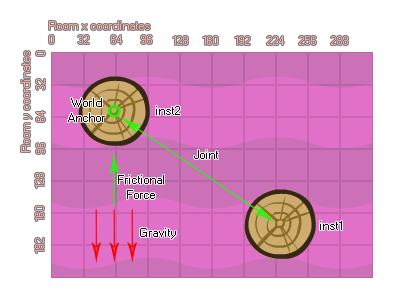# physics_joint_friction_create

## A joint for reducing the relative motion between two fixtures.

### 语法：

physics_joint_friction_create(inst1, inst2, anchor_x, anchor_y, max_force, max_torque, col)

inst1 The first instance to connect with the joint
inst2 The second instance to connect with the joint
anchor_x The x coordinate for the joint, within the game world
anchor_y The y coordinate for the joint, within the game world
max_force The maximum frictional force that will be applied
max_torque The maximum rotational force that will be applied
col Whether the two instances can collide (true) or not (false)

Returns: Index of the joint

### 描述

The friction joint is a bit different to all other joints in the physics simulation in that the connection created will not constrain the instances position or movement, but rather its speed and rotation. This works by taking the maximum input values for force and torque and applying those to the second fixture to bring the speed and angular momentum down to the same values as that of the first instance. So, if you have a stationary instance and a moving instance then connect them with a friction joint, the moving instance will gradually slow down until it too is stationary. If both instances were moving then the second instance will have its movement speed modified to match that of the first instance.As with most other joints, you supply the instances to join together, as well as the position for the joint to be created at within the room. You then supply the maximum force and maximum torque (directional and rotational friction), and if you set the "col" value to true then the two instances can interact and collide with each other but only if they have collision events, however if it is set to false, they will not collide no matter what.

### 例如：

var mainFixture, o_id;
mainFixture = physics_fixture_create();
physics_fixture_set_circle_shape(mainFixture, sprite_get_width(sprite_index) / 2);
o_id = instance_create_layer(x+300, y, "Instances", obj_Rudder);
physics_fixture_bind(mainFixture, id);
physics_fixture_bind(mainFixture, o_id);
physics_joint_friction_create(id, o_id, x, y, 10, 0.3, true);
physics_fixture_delete(mainFixture);

The above code will create a fixture then bind it to two instances, before connecting them both using a friction joint.